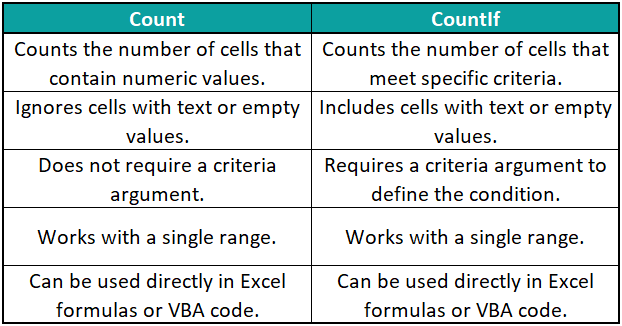# VBA CountIfArticle byExcelMojo Team## What is Excel VBA CountIf?

The Excel VBA CountIf function counts the number of cells in a range that meets a specific condition or criteria. It is like the Excel worksheet function COUNTIF, but it is used within VBA code to perform calculations and manipulate data programmatically.

Consider an example where you must find the number of apples in a column. It can be done by implementing the VBA CountIf range variable as seen below:

This example prints the number of Apples present in the table in the Immediate tab.

###### Key Takeaways
• COUNTIF in VBA is used to count cells that meet specific criteria in Excel through VBA code.
• It is accessed through the Application.WorksheetFunction object.
• COUNTIF takes two arguments: the range of cells to evaluate and the criteria to apply.
• It allows for programmatically performing calculations and data manipulations.
• COUNTIF in VBA is useful for automating data analysis and processing within VBA macros.

### Syntax of VBA CountIf

The syntax for the CountIf function in VBA is as follows:

Application.WorksheetFunction.CountIf(range, criteria)

Where:

• range is the range of cells in which you want to count the occurrences.
• criteria are the conditions that the cells must meet to be counted.

Note: CountIf is part of the WorksheetFunction object in the Application class. Hence, VBA CountIf is always called with the prefix “Application.WorksheetFunction.”

### How to Use VBA CountIf?

To utilize VBA CountIf not blank function in Excel VBA, follow the steps shown below.

1. Go to the “Developer” section in the toolbar and click the “Visual Basic” option. Now, the VBA Editor opens to add functions and sub procedures. Then, click the “Insert” and “Module” buttons to create a new module or blank page.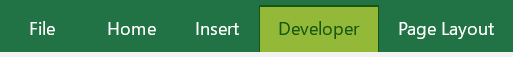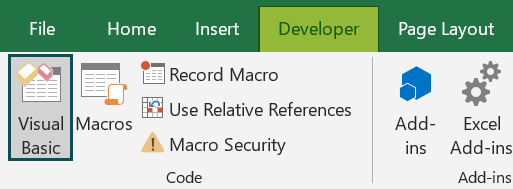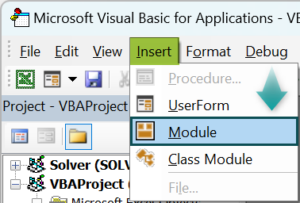2. Initialize a subroutine to print the number of cells with the date “01-01-2023” in them. The table is shown below: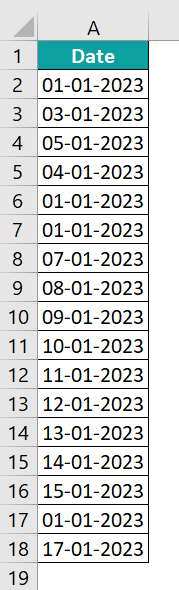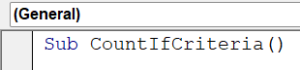3. Define variables count, rng, and criteria. The variables “count” stores the value given by the VBA CountIf Array function, “rng” stores the range of cells checked by the VBA CountIf Range Variable, and “criteria” is initialized as “Date” since our criteria are in date format.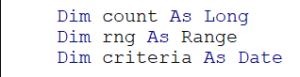4. Set the range of the table.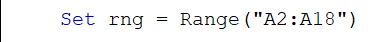5. Set the criteria for the CountIf function.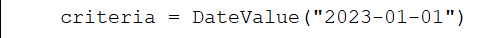6. Set the count variable as the count value of the number of “01-01-2023” between the cell range A2-A18.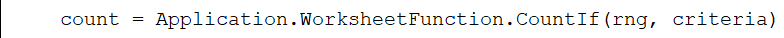The CountIf function is part of the WorksheetFunction class. Hence while defining it we call it with the prefix “Application.WorksheetFunction.CountIf(rng, criteria)”.

7. Print the count variable in the Immediate tab.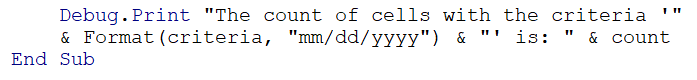Code:

Sub CountIfCriteria()
Dim count As Long
Dim rng As Range
Dim criteria As Date
Set rng = Range(“A2:A18”)
criteria = DateValue(“2023-01-01”)
count = Application.WorksheetFunction.CountIf(rng, criteria)
Debug.Print “The count of cells with the criteria ‘” & Format(criteria, “mm/dd/yyyy”) & “‘ is: ” & count
End Sub

8. Print the count value by pressing the F5 button or the run button present on the VBA toolbar.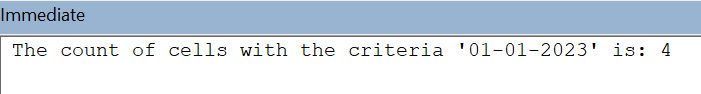### Examples

Let us look at some examples of how to use the VBA CountIf function in Excel VBA.

#### Example #1

Consider an example where we need to print the count of a specific Product in a company based on the number of available products. It can be done using VBA CountIf Multiple Criteria.

• Step 1: Define a sub-procedure which prints the count of “Product A” when its quantity is greater than 7.
• Step 2: Define variables count and rng. The variable “count” stores the count value returned by the VBA CountIf Multiple Criteria, and “rng” stores the cell range in which the CountIf conditions are checked.
• Step 3: Set the VBA range as the range of the table. It checks whether the product is “Product A” and the quantity.
• Step 4: Assign “count” as the value returned by VBA CountIf Multiple Criteria.

Note:

Application.WorksheetFunction.CountIfs(rng, “Product A”, rng.Offset(0, 1), “>7”) utilizes the CountIfs function in the WorksheetFunction object to count the number of cells in a range that meet specific criteria. Here are its components:

• rng: Represents the range of cells where the count will be performed.
• “Product A”: Specifies the criteria for the first condition. It counts the cells in rng that contain the value “Product A”.
• rng.Offset(0, 1): Represents a range offset by one column from rng. It is used for the second condition.
• “>7”: Specifies the criteria for the second condition. It counts the cells in the offset range that has a value greater than 7.

The CountIfs function calculates the count of cells that satisfy both conditions simultaneously.

• Step 5: Print the “count” variable in the immediate table.

Code:

Sub CountIfMultipleConditions()
Dim count As Long
Dim rng As Range
Set rng = Range(“C2:D18”)
count = Application.WorksheetFunction.CountIfs(rng, “Product A”, rng.Offset(0, 1), “>7”)
Debug.Print “The count is: ” & count
End Sub

• Step 6: Print the above VBA code. The output is printed in the immediate tab.

#### Example #2

Take an example where you need to count the number of students who have scored above 85 in their exams in the table below:

• Step 1: Initialize a subroutine to count the number of students who scored above the threshold value.
• Step 2: Initialize the variables count, rng and threshold. The variable “count” is used to store the count of students who scored above the “threshold” defined by the user.
• Step 3: Initialize the range value to check for the numbers greater than the threshold value.
• Step 4: Define the threshold value for the student’s table.
• Step 5: Define the count variable by performing VBA CountIf between the given range.

CountIf then compares the values in the range and checks if they are above 85. If so, the counter is incremented. If not, the counter is not incremented.

• Step 6: Print the output using the MsgBox function.

Code:

Sub CountStudentsAboveThreshold()
Dim count As Long
Dim rng As Range
Dim threshold As Long
Set rng = Range(“B2:B20”)
threshold = 85
count = Application.WorksheetFunction.CountIf(rng, “>85”)
MsgBox “The number of students who scored above ” & threshold & ” is: ” & count
End Sub

• Step 7: Print the above code. The output is printed in a message box.

### Arrive Result with Variables

Another way of performing VBA CountIf Multiple criteria is by defining them with multiple variables and calling them in the CountIf function. Let’s see how to do that.

Consider an example where the quantity of certain electronic items sold on a particular day is given. We must print the count of the number of computers sold which is greater than 5.

• Step 1: Initialize a subroutine to count the number of sales of computers which have sold above 5.
• Step 2: Initialize the variables count, rng and threshold, criteria1 and criteria2. The variable “count” is used to store the count of sales of “criteria1” greater than “criteria2.” The variable “rng” is defined as the range of the table.
• Step 3: Initialize the range value to check the table for the criteria.
• Step 4: Initialize the variables to define the criteria for the VBA CountIf Multiple Variables.
• Step 5: Define the count variable by performing VBA CountIf using the criteria between the given range.

Code:

Sub CountIfMultipleConditionsWithVariables()
Dim count As Long
Dim rng As Range
Dim criteria1 As String
Dim criteria2 As String
Set rng = Range(“A2:B10”)
criteria1 = “Computer”
criteria2 = “>5”
count = Application.WorksheetFunction.CountIfs(rng, criteria1, rng.Offset(0, 1), criteria2)
MsgBox “The count is: ” & count
End Sub

• Step 6: Press “F5” to print the output. It will be displayed in a message box.

### Important Things to Note

• Ensure that you provide the correct syntax when using the COUNTIF function. The range and criteria arguments should be accurate and properly formatted.
• The criteria you apply can be a specific value, a cell reference, a wildcard pattern, or a logical expression.
• You can use wildcards such as asterisks (*) and question marks (?) in your criteria to represent unknown or variable characters in the cell values you want to count.
• If you plan to copy the COUNTIF formula to other cells, use absolute references (e.g., \$A\$1:\$A\$10) to lock the range references. It ensures that the formula always refers to the correct range.

1. Why is VBA COUNTIF not working?

Check if the range and criteria arguments are correctly specified.
Ensure that the range reference is valid and covers the desired data range.
Verify that the criteria are in the correct format and match the data type of the cells being evaluated.
Watch out for any leading or trailing spaces in the criteria that might cause a mismatch.
Check if there are any hidden cells or filtered data that may affect the count.
Make sure you are using the correct syntax and function name (COUNTIF, not COUNTIFS).
Check if there are any error values or non-numeric/non-text values in the range that could affect the count.
Verify that the WorksheetFunction object is correctly used to access the COUNTIF function in VBA.

2. What is the difference between Countif and Countifs in VBA?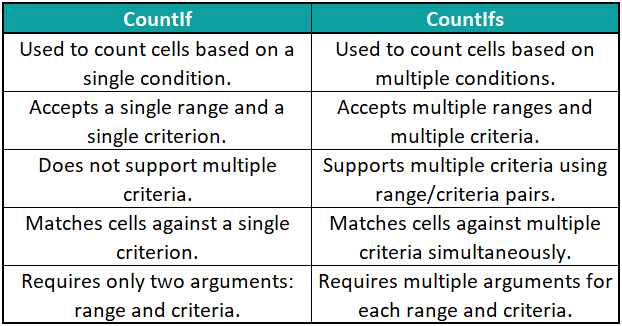3. What is the difference between Count and Countif in VBA?